Courses

# Lagrangian and Hamiltonian Formalism and equations of motion (Part - 3) - CSIR-NET Physical Sciences Physics Notes | EduRev

## Physics : Lagrangian and Hamiltonian Formalism and equations of motion (Part - 3) - CSIR-NET Physical Sciences Physics Notes | EduRev

The document Lagrangian and Hamiltonian Formalism and equations of motion (Part - 3) - CSIR-NET Physical Sciences Physics Notes | EduRev is a part of the Physics Course Physics for IIT JAM, UGC - NET, CSIR NET.
All you need of Physics at this link: Physics

Don’t plug Equations of Motion into the Lagrangian!

When we have a Lagrangian with an ignorable coordinate, say θ, and therefore a conjugate momentum Pθ which is conserved and can be considered a constant, we are able to reduce the problem to one involving one fewer degrees of freedom. That is, one can substitute into the other differential equations the value of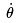in terms of Pθ and other degrees of freedom, so that θ and its derivatives no longer appear in the equations of motion. For example, consider the two dimensional isotropic harmonic oscillator,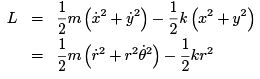in polar coordinates. The equations of motion are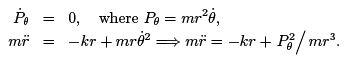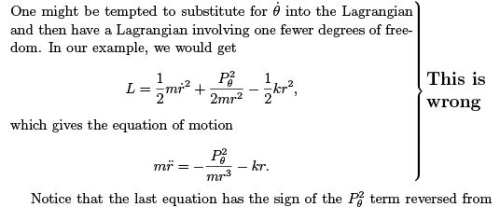the correct equation. Why did we get the wrong answer? In deriving the Lagrange equation which comes from varying r, we need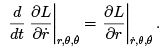But we treated Pθ as fixed, which means that when we vary r on the right hand side, we are not holdingfixed, as we should be. While we often write partial derivatives without specifying explicitly what is being held fixed, they are not de ned without such a speci cation, which we are expected to understand implicitly. However, there are several examples in Physics, such as thermodynamics, where this implicit understanding can be unclear, and the results may not be what was intended.

2.7 Velocity-dependent forces

We have concentrated thus far on Newtonian mechanics with a potential given as a function of coordinates only. As the potential is a piece of the Lagrangian, which may depend on velocities as well, we should also entertain the possibility of velocity-dependent potentials. Only by considering such a potential can we possibly nd velocity-dependent forces, and one of the most important force laws in physics is of that form. This is the Lorentz force9 on a particle of charge q in the presence of electromagnetic elds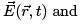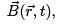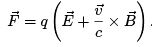(2.18)

If the motion of a charged particle is described by Lagrangian mechanics with a potential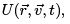Lagrange’s equation says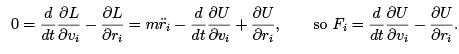We want a force linear in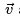and proportional to q, so let us try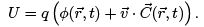Then we need to have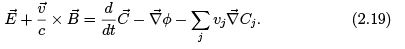The first term is a stream derivative evaluated at the time-dependent position of the particle, so, as in Eq. (2.5),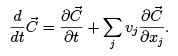The last term looks like the last term of (2.19), except that the indices on the derivative operator and on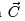have been reversed. This suggests that these two terms combine to form a cross product. Indeed, noting (A.17) that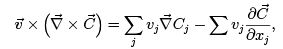we see that (2.19) becomes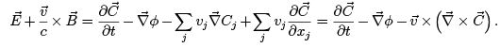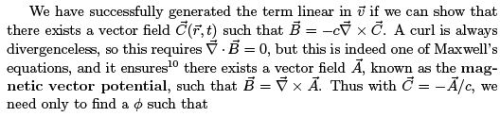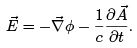Once again, one of Maxwell’s laws,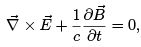guarantees the existence of φ, the electrostatic potential, because after inserting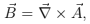this is a statement that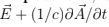has no curl, and is the gradient of something.

Thus we see that the Lagrangian which describes the motion of a charged particle in an electromagnetic eld is given by a velocity-dependent potential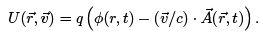Note, however, that this Lagrangian describes only the motion of the charged particle, and not the dynamics of the eld itself.

Arbitrariness in the Lagrangian :

In this discussion of nding the Lagrangian to describe the Lorentz force, we used the lemma that guaranteed that the divergenceless magnetic field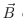can be written in terms of some magnetic vector protential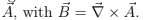But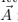is not uniquely specified by; in fact , if a change is made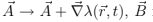is unchanged becaurse the cirl of a gradient vanished. The electric field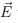will be changed by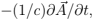however, unless we also make a change in electrostatic prtential,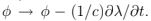if we do, we have completely unchanged electromagnetic fields, which is where the physics lies. This change in the potentials,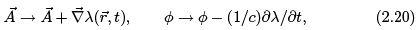is known as a gauge transformation, and the invariance of the physics under this change is known as gauge invariance. Under this change, the potential U and the Lagrangian are not unchanged,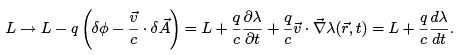We have here an example which points out that there is not a unique Lagrangian which describes a given physical problem, and the ambiguity is more that just the arbitrary constant we always knew was involved in the potential energy. This ambiguity is quite general, not depending on the gauge transformations of Maxwell elds. In general, if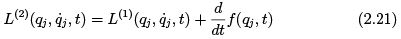then L(1) and L(2) give the same equations of motion, and therefore the same physics, for qj (t). While this can be easily checked by evaluating the Lagrange equations, it is best understood in terms of the variation of the action. For any path q(t) between qjI at t = tto qjF at t = t, the two actions are related byThe variation of path that one makes to nd the stationary action does not change the endpoints qjF and qjI , so the difference S (2) − S (1) is a constant independent of the tra jectory, and a stationary tra jectory for S (2) is clearly stationary for S (1) as well.
The conjugate momenta are affected by the change in Lagrangian, however, because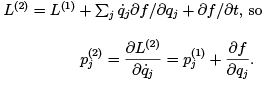This ambiguity is not usually mentioned in elementary mechanics, because if we restict our attention to Lagrangians consisting of canonical kinetic energy and potentials which are velocity-independent, a change (2.21) to a Lagrangian L(1) of this type will produce an L(2) which is not of this type, unless f is independent of position q and leaves the momenta unchanged. That is, the only f which leaves U velocity independent is an arbitrary constant.

Dissipation Another familiar force which is velocity dependent is friction.
Even the "constant" sliding friction met with in elementary courses depends on the direction, if not the magnitude, of the velocity. Friction in a viscous medium is often taken to be a force proportional to the velocity,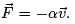We saw above that a potential linear in velocities produces a force perpendicular to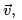, and a term higher order in velocities will contribute a force that depends on acceleration. This situation cannot handled by Lagrange’s equations. More generally, a Lagrangian can produce a force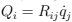with antisymmetric Rij , but not for a symmetric matrix. An extension to the Lagrange formalism, involving Rayleigh’s dissipation function, can handle such a case.

Exercises

2.7. VELOCITY-DEPENDENT FORCES 59

2.1 (Galelean relativity): Sally is sitting in a railroad car observing a system of particles, using a Cartesian coordinate system so that the particles are at positions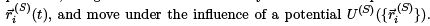Thomas is in another railroad car, moving with constant velocity ~u with respect to Sally, and so he describes the position of each particle as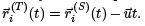Each takes the kinetic energy to be of the standard form in his system, i.e.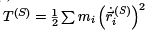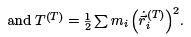(a) Show that if the potential function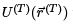to be the same as Sally’s at the same physical points,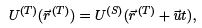(2.22)

then the equations of motion derived by Sally and Thomas describe the same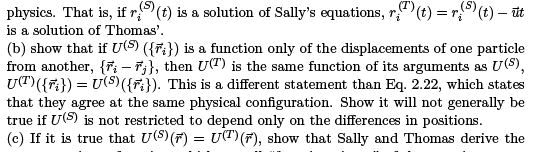same equations of motion, which we call \form invariance" of the equations.

(d) Show that nonetheless Sally and Thomas disagree on the energy of a particular physical motion, and relate the difference to the total momentum. Which of these quantities are conserved?

2.2 In order to show that the shortest path in two dimensional Euclidean space is a straight line without making the assumption that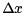does not change sign along the path, we can consider using a parameter λ and describing the path by two functions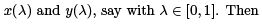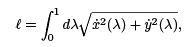where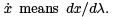This is of the form of a variational integral with two variables. Show that the variational equations do not determine the functions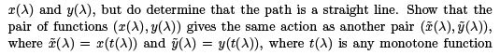mapping [0; 1] onto itself. Explain why this equality of the lengths is obvious in terms of alternate parameterizations of the path. [In eld theory, this is an example of a local gauge invariance, and plays a ma jor role in string theory.]

2.3 Consider a circular hoop of radius R rotating about a vertical diameter at a xed angular velocity Ω. On the hoop there is a bead of mass m, which slides without friction on the hoop. The only external force is gravity. Derive the Lagrangian and the Lagrange equation using the polar angle θ as the unconstrained generalized coordinate. Find a conserved quantity, and nd the equilibrium points, for which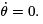. Find the condition on Ω such that there is an equilibrium point away from the axis.

2.4 Early steam engines had a feedback device, called a governor, to automatically control the speed. The engine rotated a vertical shaft with an angular velocity Ω proportional to its speed. On opposite sides of this shaft, two hinged rods each held a metal weight, which was attached to another such rod hinged to a sliding collar, as shown.

As the shaft rotates faster, the balls move outwards, the collar rises and uncovers a hole, releasing some steam. Assume all hinges are frictionless, the rods massless, and each ball has mass m1 and the collar has mass m2.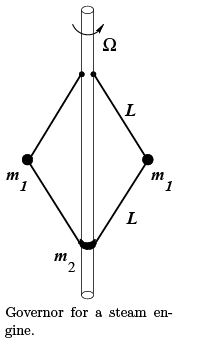(a) Write the Lagrangian in terms of the generalized coordinate θ.

(b) Find the equilibrium angle θ as a function of the shaft angular velocity Ω. Tell whether the equilibrium is stable or not.

2.5 A transformer consists of two coils of conductor each of which has an inductance, but which also have a coupling, or mutual inductance.

2.7. VELOCITY-DEPENDENT FORCES

If the current ﬂowing into the upper posts of coils A and B are IA(t)and IB (t) respectively, the voltage difference or EMF across each coil is Vand VB respectively, where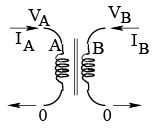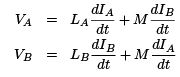Consider the circuit shown, two capacitors coupled by a such a transformer, where the capacitances are CA and CB respectively, with the charges q1 (t)and q2 (t) serving as the generalized coordinates for this problem. Write down the two second order differential equations of "motion" for q1 (t) and q2 (t), and write a Lagrangian for this system.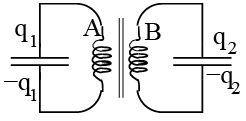2.6 A cylinder of radius R is held horizontally in a xed position, and a smaller uniform cylindrical disk of radius a is placed on top of the rst cylinder, and is released from rest. There is a coefficient of static friction μs and a coefficient of kinetic friction μks for the contact between the cylinders. As the equilibrium at the top is unstable, the top cylinder will begin to roll on the bottom cylinder.

(a) If μs is sufficiently large, the small disk will roll until it separates from the fixed cylinder. Find the angle θ at which the separation occurs, and nd the minimum value of μs for which this situation holds.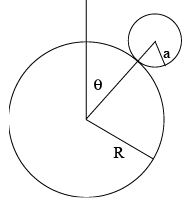A small cylinder rolling on a xed larger cylinder.

(b) If μs is less than the minimum value found above, what happens differently, and at what angle θ does this different behavior begin?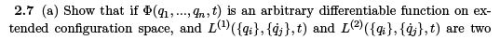Lagrangians which differ by the total time derivative of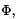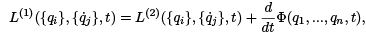show by explicit calculations that the equations of motion determined by L(1) are the same as the equations of motion determined by L(2). (b) What is the relationship between the momenta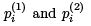determined by these two Lagrangians respectively.

2.8 A particle of mass m1 moves in two dimensions on a frictionless horizontal table with a tiny hole in it. An inextensible massless string attached to m1 goes through the hole and is connected to another particle of mass m2, which moves vertically only. Give a full set of generalized unconstrained coordinates and write the Lagrangian in terms of these. Assume the string remains taut at all times and that the motions in question never have either particle reaching the hole, and there is no friction of the string sliding at the hole.
Are there ignorable coordinates? Reduce the problem to a single second order differential equation. Show this is equivalent to single particle motion in one dimension with a potential V (r), and nd V (r).

2.9 Consider a mass m on the end of a massless rigid rod of length ℓ the other end of which is free to rotate about a fi xed point. This is a spherical pendulum.
Find the Lagrangian and the equations of motion.

2.10 (a) Find a differential equation for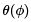for the shortest path on the surface of a sphere between two arbitrary points on that surface, by minimizing the length of the path, assuming it to be monotone in φ. (b) By geometrical argument (that it must be a great circle) argue that the path should satisfy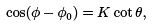and show that this is indeed the solution of the differential equation you derived.

2.11 Consider some intelligent bugs who live on a turntable which, according to inertial observers, is spinning at angular velocity ω about its center. At any one time, the inertial observer can describe the points on the turntable with polar coordinates r, φ. If the bugs measure distances between two ob jects at rest with respect to them, at in nitesimally close points, they will find

2.7. VELOCITY-DEPENDENT FORCES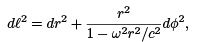because their metersticks shrink in the tangential direction and it takes more of them to cover the distance we think of as rdφ, though their metersticks agree with ours when measuring radial displacements.
The bugs will declare a curve to be a geodesic, or the shortest path between two points, if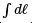is a minimum. Show that this requires that r(φ) satis es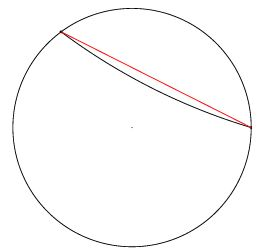Straight lines to us and to the bugs, between the same two points.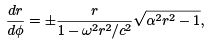where α is a constant.

2.12 Hamilton’s Principle tells us that the motion of a particle is determined by the action functional being stationary under small variations of the path Γ in extended con guration space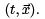The unsymmetrical treatment of t and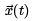is not suitable for relativity, but we may still associate an action with each path, which we can parameterize with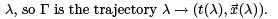In the general relativistic treatment of a particle’s motion in a gravitational eld, the action is given by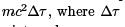is the elapsed proper time,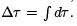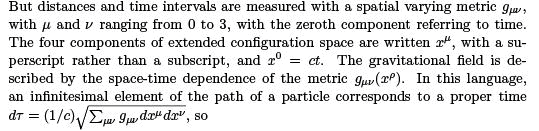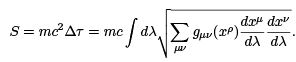(a) Find the four Lagrange equations which follow from varying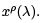(b) Show that if we multiply these four equations by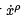and sum on ρ,weget an identity rather than a differential equation helping to determine the functions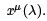Explain this as a consequence of the fact that any path has a length unchanged by a reparameterization of the path,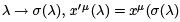(c) Using this freedom to choose λ to be τ , the proper time from the start of the path to the point in question, show that the equations of motion are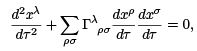and nd the expression for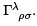2.13 (a): Find the canonical momenta for a charged particle moving in an electromagnetic eld and also under the inﬂuence of a non-electromagnetic force described by a potential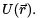(b): If the electromagnetic eld is a constant magnetic field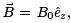with no electric eld and with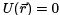, what conserved quantities are there?

Offer running on EduRev: Apply code STAYHOME200 to get INR 200 off on our premium plan EduRev Infinity!

159 docs

,

,

,

,

,

,

,

,

,

,

,

,

,

,

,

,

,

,

,

,

,

;